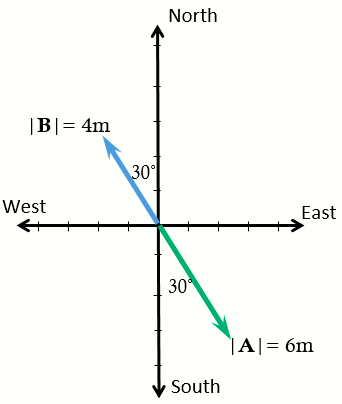# Vector A has a magnitude of 6.0 m and points 30 degree east of south. Vector B has a magnitude of...

## Question:

Vector A has a magnitude of 6.0 m and points {eq}30^{\circ} {/eq} east of south. Vector B has a magnitude of 4.0 m and points {eq}30^{\circ} {/eq} west of north. The resultant vector A + B is given by:

A) 2.0 m at an angle of {eq}30^{\circ} {/eq} east of south.

B) 10.0 m at an angle of {eq}60^{\circ} {/eq} east of south.

C) 1.0 m at an angle of {eq}60^{\circ} {/eq} north of west.

D) 2.0 m at an angle of {eq}30^{\circ} {/eq} north of west.

E) 10.0 m at an angle of {eq}60^{\circ} {/eq} north of west.

## Vector

Vector quantities or vectors are quantities that have both direction and magnitude such as velocity, acceleration, and force. Vector quantities are mathematical objects that are represented by arrows or ordered set of numbers.

The vectors in the problem are shown in the figure below.From the figure, we can see that both vectors lie on the same line, so we can call vector A as {eq}\mathbf{A} = - 6.0\ m {/eq} and vector B as {eq}\mathbf{B} = 4.0\ m {/eq}.

The resultant vector is the algebraic sum of {eq}\mathbf{A} {/eq} and {eq}\mathbf{B} {/eq}.

{eq}\displaystyle \begin{align*} \mathbf{A} + \mathbf{B} &= (-6.0\ m) + 4.0\ m \\ &= -2.0\ m \end{align*} {/eq}

The resultant vector has the same sign as {eq}\mathbf{A} {/eq}, so they point in the same direction. This works because our vectors A and B lie along the same line. And the magnitude is given by {eq}| -2.0\ m| = 2.0\ m {/eq}.

Therefore, the resultant vector is {eq}\boxed{ \text{ A) 2.0 m at an angle of 30}^ {\circ} \text{ east of south.}} {/eq}